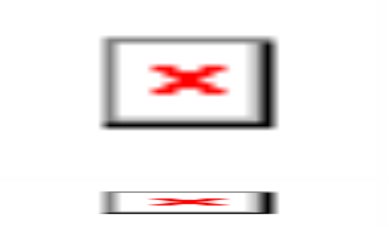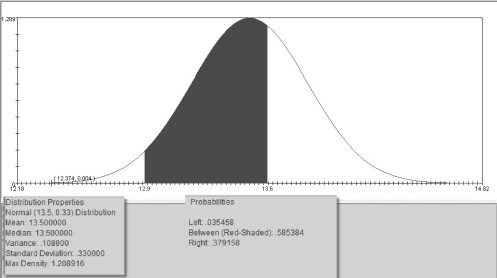• FAQ
• Contact/ Homework Answers / Statistics / 1. Determine whether the variable would best be modeled as
Not my Question
Flag Content

# Question

1. Determine whether the variable would best be modeled as continuous or discrete:  The number of cups dispensed from a beverage vending machine during a 24-hour period.

a.Continuous

b.Discrete

2. Determine whether the variable would best be modeled as continuous or discrete:  The temperature of a cup of coffee dispensed from a beverage vending machine, taken four times during a 24-hour period.

a.Continuous

b.Discrete

3. At a course in public speaking, the instructor always gives an opening speech that lasts between fifteen and eighteen minutes.  The length of the speech can be modeled by a uniform distribution, that is, the speech is just as likely to last fifteen minutes as it is to last eighteen minutes.  The probability density curve is shown below.  What is the probability that the speech will last sixteen minutes or more?  What is the probability that the speech will last between eighteen and nineteen minutes?a.Can’t be determined with the given information

b.0.50; 0.75

c.0.75; 0.25

d.0.50; 0.25

4. A box containing recipes from five categories is dropped so that the recipe cards are thoroughly mixed up.  The following table shows the possible categories and the associated probability for a recipe randomly chosen.  Does the table represent a probability distribution?

CategoryProbability

Main Dish0.421

Appetizer0.210

Desert0.103

Vegetable0.093

a.Yes

b.No

c.Can’t be determined with the given information

Use the following information for questions (5)-(7).  Male players at the high school, college and professional ranks use a regulation basketball that weighs 22.0 ounces with a standard deviation of 1.0 ounce.  Assume that the weights of basketballs are approximately normally distributed.

5. Roughly what percentage of regulation basketballs weigh more than 23.1 ounces?  Round to the nearest tenth of a percent.

a.Roughly 15.1% of the basketballs will weigh more than 23.1 ounces.

b.Roughly 42.3% of the basketballs will weigh more than 23.1 ounces.

c.Roughly 36.4% of the basketballs will weigh more than 23.1 ounces.

d.Roughly 13.6% of the basketballs will weigh more than 23.1 ounces.

6.

If a regulation basketball is randomly selected, what is the probability that it will weigh between 19.5 and 22.5 ounces?  Round to the nearest thousandth. a.0.547

b.0.315

c.0.685

d.0.723

7. Would it be unusual to randomly select a regulation basketball and find that it weighs 23.75 ounces?

a.Yes, this would be unusual.

b.No, this would not be unusual.

Chapter 6 Test B       6-3

8. Suppose that weights of cans of Benneke brand peaches have a population mean of 13.5 ounces and a population standard deviation of 0.33 ounces and are approximately normally distributed.  Which of the following statements are correct?  Choose the best statement.a.Approximately 95% of all Benneke brand canned peaches will weigh between 12.85 ounces and

14.15 ounces.

b.The probability that a randomly selected can of Benneke peaches will weigh between 12.9 ounces and 13.6 ounces is approximately 0.585.

c.About 4% of all cans of Benneke peaches will weigh less than 12.9 ounces

d.All of the above statements are true.

Use the following information for questions (9) - (11).  The average travel time to work for a person living and working in Kokomo, Indiana is 17 minutes.  Suppose the standard deviation of travel time to work is 4.5 minutes and the distribution of travel time is approximately normally distributed.

9. Approximately what percentage of people living and working in Kokomo have a travel time to work that is less than 15.5 minutes?  Round to the nearest whole percent.

a.37%

b.63%

c.25%

d.None of the above.

10. Which of these statements is asking for a probability?

a.What percentage of people living and working in Kokomo has a travel time to work that is between thirteen and fifteen minutes?

b.If 15% of people living and working in Kokomo have travel time to work that is below a certain number of minutes, how many minutes would that be?

## Solution 5 (1 Ratings )

Solved
Statistics 3 Months Ago 7 Views